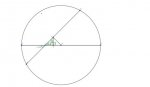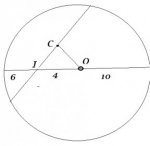# Diameter and chord in a circle

#### kaloyankolev

##### New member
Hi,

Can someone help me with the following problem:

A chord intersects the diameter at an angle 45 degrees and divide it into two segments with lengths 6 cm and 14 cm. Find the distance between the center of the circle and the middle of the chord.

Sorry for my English. It isn't good enough to describe math problems but I did my best. I made a drawing and I hope that you will understand me. I've thought for two hours but I can't understand how to solve it.#### Dr.Peterson

##### Elite Member
A chord intersects the diameter at an angle 45 degrees and divide it into two segments with lengths 6 cm and 14 cm. Find the distance between the center of the circle and the middle of the chord.

Sorry for my English. It isn't good enough to describe math problems but I did my best. I made a drawing and I hope that you will understand me. I've thought for two hours but I can't understand how to solve it.

View attachment 10919
I understand the problem quite well.

First step: Can you find the distance from the center of the circle to the intersection point?

Second step: Do you see that the triangle you drew is a right triangle?

Third step: What does this 45-45-90 triangle tell you about the distance from the center to the chord?

EDIT: To be clearer, I can't see the names of the points in your picture, but the triangle I referred to is the larger one, which you divided into two smaller right triangles, whose vertices are the center of the circle, the midpoint of the chord, and the intersection of the chord and the diameter.

Last edited:

#### Denis

##### Senior Member
A chord intersects the diameter at an angle 45 degrees and divide it into two segments with lengths 6 cm and 14 cm. Find the distance between the center of the circle and the middle of the chord.

Sorry for my English. It isn't good enough to describe math problems but I did my best. I made a drawing and I hope that you will understand me. I've thought for two hours but I can't understand how to solve it.
Your English is quite good...better than many English studentsLast edited:

#### pka

##### Elite Member
A chord intersects the diameter at an angle 45 degrees and divide it into two segments with lengths 6 cm and 14 cm. Find the distance between the center of the circle and the middle of the chord.
I understand the problem quite well.
Third step: What does this 45-45-90 triangle tell you about the distance from the center to the chord?
EDIT: To be clearer, I can't see the names of the points in your picture, but the triangle I referred to is the larger one, which you divided into two smaller right triangles, whose vertices are the center of the circle, the midpoint of the chord, and the intersection of the chord and the diameter.Like Prof Peterson, I cannot read your image. Here is mine, but you must bare with me it is by no means drawn accurately. $$\displaystyle O$$ is the circle's center, $$\displaystyle \overline{OC}$$ is the perpendicular bisector of the chord.(I said the diagram is not accurately)
There is a well known theorem: the perpendicular bisector of the chord of a circle passes thru the centre.
Because $$\displaystyle m(\angle OIC)=\frac{\pi}{4}$$ then that means $$\displaystyle m(\angle IOC)=\frac{\pi}{4}$$.
So the $$\displaystyle \Delta ICO$$ is an isosceles right triangle. Use its the fact that its hypotenuse if $$\displaystyle 4$$ units to find the length of $$\displaystyle \overline{OC}$$.
Now you must supply details.

Last edited: